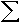## EasyWorksheet's Online Book/Reference Guide Example:

Students have access to an online book/reference guide for most subjects. Each page covers just one objective. That way the students can focus on what they are learning! The guide is interactive (students have a few problems to try on each page -- and they are told instantly if they are correct or wrong. We also break down each topic so that students can understand how to do the problems. Unlike textbooks that go more into history or go above the heads of the students, we focus on the important parts (how to do the problems) so that students can really "get it"!

## Finding Summations of Arithmetic SeriesFirst, remember that ARITHMETIC means ADDITION, and SERIES is a SUM of a list of numbers. The SUMMATION sign,, is sigma from the Greek Alphabet. Anytime you see, think SUM!

### New Vocabularymeans SUM!

## What does it mean?

Whenever you are faced with a summation sign, there are numbers on the bottom and top of it like so:
 5n=-3 3n - 2

The value on the bottom is where we start. So we'll start at n = -3. And we end up at the value on the top. So we'll end at n = 5. The function is written in the middle. It really doesn't matter what type of function you have!

In order to solve this problem, we'll make a chart. One column of the chart will be n (our variable) and the other side will be 3n - 2 (the function). We simply fill in our n values to 3n - 2 in order to get the other value. For instance, we can plug in -1 for n. Hence, 3n - 2 = 3(-1) - 2 = -3 - 2 = -5. Notice that when n = -1, we have -5 under 3n - 2.

```n    3n - 2
-----------
-3    -11
-2    -8
-1    -5 ← See the -5 here?
0    -2
1    1
2    4
3    7
4    10
5    13
```

Finally, we SUM up all the numbers on the right:
-11 + -8 + -5 + -2 + 1 + 4 + 7 + 10 + 13 = 9.

### This is too much work!

Well.. There is a formula!
```What happens when we add -11 + 13? (we get 2)
-11 + -8 + -5 + -2 + 1 + 4 + 7 + 10 + 13
\____________________________________/

And what about -8 + 10? (2)
-11 + -8 + -5 + -2 + 1 + 4 + 7 + 10 + 13
\_________________________/

And -5 and 7? (2) (see a pattern yet?)
-11 + -8 + -5 + -2 + 1 + 4 + 7 + 10 + 13
\________________/

-11 + -8 + -5 + -2 + 1 + 4 + 7 + 10 + 13
\_______/

```
Then notice that 1 and 1 (pairing 1 with itself) gives 2 as well?

Since there are 9 values total, the total number of groupings is 4 1/2 = 9/2 = the number of values divided by 2!

And we know that the sum is 9/2(-11 + 13)

In other words, the sum of the first n terms is:
Sn = n/2(first term + last term)

### Example #13n=0 5 - 2(n-1) =
In this case, our chart will go from 0 to 3:
```n        5 - 2(n-1)
-------------------
0        7
1        5
2        3
3        1
```
Then we'll add: 7 + 5 + 3 + 1 = 16 ← our answer!

### Example #2

 229n=-30 2n + 5 =

In this case, it would be easier to use the formula. So we'll begin by finding the first and last terms:
The first term (filling in n = -30) :
2(-30) + 5 = -60 + 5 = -55
The last term (filling in n = 229) :
2(229) + 5 = 458 + 5 = 463

Next, subtract the upper and lower bounds and add 1 to get the number of terms:
(229) - (-30) + 1 = 229 + 30 + 1 = 260.

Now, we'll fill in the formula:
Sn = n/2(first term + last term)
Sn = (260)/2(-55 + 463) = (130)(408) = 53040

Aren't you glad we used the formula? I wouldn't want to make a chart with 260 entries!! (Warning: Only use the formula in the case of ARITHMETIC sequences!! )

### Test Taking Strategies

For multiple choice tests, the best thing you can do is remember thatmeans SUM. That way no matter what function you are given, you can always find the sum in a chart!

## Review What You Learnedmeans SUM!
The formula for summing an ARITHMETIC sequence (linear function) is
Sn = n/2(first term + last term)

### Example #3 (You try it!)

 5n=2 n2 =Hint: This function is NOT arithmetic!!

### Example #4 (You try it!)

 25n=2 -7 + 2(n-1) =